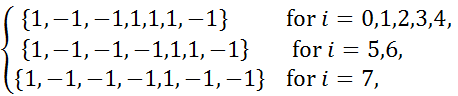Proofs of Lemma 3.2(ii) and assertion (4.27) appeared in my paper

Exact multiplicity and bifurcation curves of positive solutions of a one-dimensional Minkowski-curvature equation and its application

Shao-Yuan Huang

In this website, we provide two proofs of Lemma 3.2(ii) and assertion (4.27).

(i)              Proof of Lemma 3.2(ii)  Please click this link to read this proof.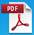(ii)           Proof of assertion (4.27)  Please click this link to read this proof.Notice that in the proof of assertion (4.27), there are many complex computations such as (1.10)-(1.11), (1.14)-(1.15), and (1.18)-(1.19). To verify them, codes and outputs are given in following links. Readers can run these codes by Maple 16 to check the computations. These computations which are carried out by computer are based on symbolic and exact integer computations.

Varifications

(i)              In (1.10) and (1.11),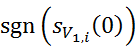=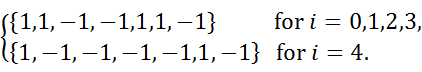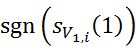=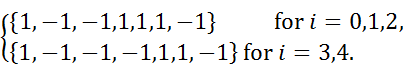(ii)           In (1.14) and (1.15),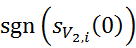=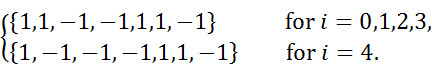=(iii)        In (1.18) and (1.19),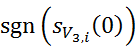=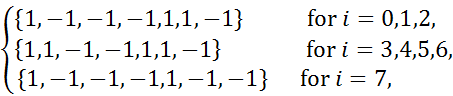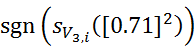=